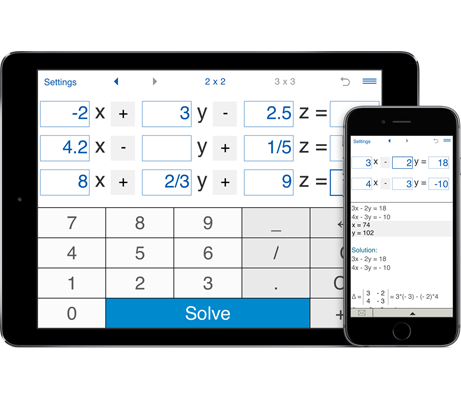# System solver by Intemodino

Intuitive calculator designed to solve systems of linear equations with two and three variables using substitution and Cramer's rule.

## How to use System Solver

#### Does System Solver show the steps? How can I print the solution?

After you enter the coefficients and press Solve the calculator shows you both - the result and detailed explanation. You may easily print the solution by sending the calculations via email and then printing them out.

#### How can I input the 3x3 system of linear equations on my iPhone? When I run the application it does not show any options to entre the system of equations with three variables.

The calculator solves systems of linear equations with two and three variables. If you use the application on your phone, you should rotate your phone to a landscape orientation to enter three equations.

#### I need to solve a system of two linear equations by substitution. Where can I choose the method?

You can choose the method from the combo box beneath the equations. You can solve 2x2 systems using either the substitution method or the Cramer's rule.

#### Can I choose the substitution method for systems of three equations?

For systems of equations with three variables, please use the Cramer's rule.

#### How can I solve systems of linear equations with four, five and more variables?

If you need to solve systems with more than three unknowns, we could recommend you our application System Solver NxN that is available for Mac and Windows computers and Android devices. Using this calculator you can solve up to 10 equations simultaneous if the system of equation has a unique solution.

#### Can I solve systems of equations with fractional coefficients?

Yes, System Solver supports integer, fractional and decimal coefficients, including negative values.

#### How can I set System Solver to show results as fractions?

No special settings are needed. After clicking the Solve button you will get results in both decimal and fractional forms.

#### Where can I find the previously solved system of equations?

You can recall the necessary problem from the calculator's history using the arrow buttons on the top of the screen.